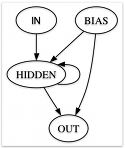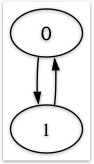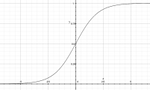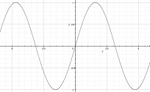Tuesday, March 30, 2010

Why I prefer Sinusoids to Sigmoids in RNNs

Recently I've been reading about Bayesian inference, and thinking about how it relates to Minimum Description Length and other similar concepts.

A key idea is that the ability to do inference over a small set of examples is, in general, a very difficult problem, and strongly dependent upon the prior probabilities of the hypothesized models matching up with the "true" model to some degree.

In the absence of any knowledge about the "true" model, things get more complicated. Some theories suggest that there should always be a bias towards simpler models, while others contradict this idea. In any case, the prior distribution over the space of hypotheses turns out to be critical to the process of inference.

Therefore, any way of gaining better intuitions about the underlying distribution of a given class of hypotheses/models is of particular interest to me.

In this post, I briefly explore some simple behavioral distributions of my own personal favorite class of models - discrete time recurrent neural networks (RNNs).

First, I randomly created a large number of extremely simple RNNs, each having the following architecture:The link weights were chosen randomly from a normal distribution with zero mean and a variance of 4.

Each randomly generated RNN was given an "impulse" input of 1.0 at the first time step, and 0.0 thereafter, and run for 10 time steps. The output of the RNN was collected and discretized to binary strings of length 10. Thus, there were exactly 1024 (210) possible unique "behaviors" that could be displayed by an RNN.

Most behaviors turned out to be very simple - all zeroes or all ones, but there were a range of other behaviors as well. I decided to plot the behaviors as a histogram. Here are the results:The original image (before scaling) is precisely 1024 by 500 pixels. Each pixel of the x-axis represents a different RNN "behavior", starting with 0000000000 at the left, and ending with 1111111111 at the right. (I considered ordering them by Gray code, but haven't implemented that just yet.) The height of the intervals in the y-axis are proportional to the natural log of the frequency of that particular behavior.

The two most immediately apparent aspects of the histogram are its symmetry and sparsity.

The edges of the histogram represent the most frequent (and simplest) behaviors - constant output of zeroes (left), and constant output of ones (right).

The two peaks nearer the center of the image occur at locations #341 and #682 along the x-axis, which, when converted to binary strings, produce the sequences 0101010101 and 1010101010, respectively. With a little thought, it is not difficult to see why these particular behaviors are so common - they can be represented by the following, extremely simple finite state machine:So it appears that these very simple RNNs are able emulate some correspondingly simple finite state machines. But, as can be seen by all of the gaps in the histogram, there are a lot of behaviors that simply never occur. As an example, sequence #589 (1001001101) never happens.

The really interesting thing I found is that these gaps in behavior are not an inherent limitation of simple RNNs, but rather, they are a limitation simple RNNs that use sigmoidal activation functions:If instead, a sinusoidal activation function is used, we get very different results!Here is another histogram of the exact same experimental setup, but this time using sinusoids as the hidden nodes of the RNNs:The difference is stunning. All 1024 binary sequences are produced!

Empirically, I have previously noticed that sinusoidal RNNs used for neuroevolution massively outperform sigmoidal ones on a wide range of tasks. I believe that now this can be at least partially explained by the fact that RNNs with sinusoidal neurons appear to have a much broader and more evenly distributed coverage of possible behaviors.

In summary: RNNs using sinusoidal hidden neurons appear to produce a more uniform prior over the space of possible dynamical models, which in turn enhances their learning capabilities over a wide range of problems.